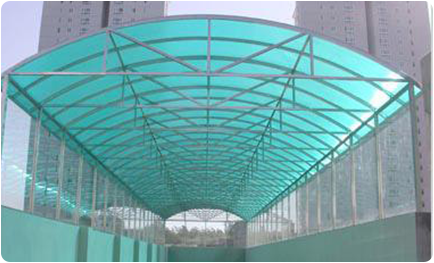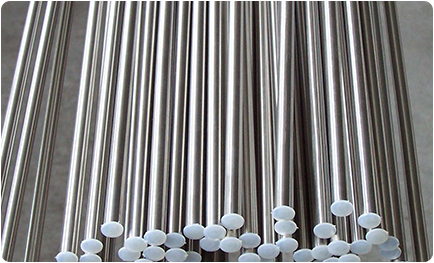8th of June ,2017

# How can i calculate stainlesss steel weight？

Theoretical Stainless Steel Weight Calculation Formula

Stainless Steel Pipes

Stainless Steel Round PipesFormula: (outer diameter - wall thickness) × wall thickness (mm) × length (m) × 0.02491

E.g.: 114mm (outer diameter) × 4mm (wall thickness) × 6m (length)

Calculation: (114-4) × 4 × 6 × 0.02491 = 83.70 (kg)

* For 316, 316L, 310S, 309S, etc., ratio=0.02507

Stainless Steel Rectangle Pipes

Formula: [(edge length + side width) × 2 /3.14- thickness] × thickness (mm) × length (m) × 0.02491

E.g.: 100mm (edge length) × 50mm (side width) × 5mm (thickness) × 6m (long)

Calculation: [(100+50)×2/3.14-5] ×5×6×0.02491=67.66 (kg)

Stainless Steel Square Pipes

Formula: (side width × 4/3.14- thickness) × thickness × length (m) × 0.02491

E.g.: 50mm (side width) × 5mm (thickness) × 6m (long)

Calculation: (50×4/3.14-5) ×5×6×0.02491 = 43.86kg

Stainless Steel Sheets/PlatesFormula: length (m) × width (m) × thickness (mm) × 7.93

E.g.: 6m (length) × 1.51m (width) × 9.75mm (thickness)

Calculation: 6 × 1.51 × 9.75 × 7.93 = 700.50kg

Stainless Steel Bars

Stainless Steel Round Bars

Formula: Dia(mm)×Dia(mm)×Length(m)×0.00623

E.g.: Φ20mm(Dia.)×6m (Length)

Calculation: 20 × 20 × 6 × 0.00623 = 14.952kg

*For 400 series stainless steel, ratio=0.00609

Stainless Steel Square Bars

Formula: side width (mm) × side width (mm) × length (m) × 0.00793

E.g.: 50mm (side width) × 6m (length)

Calculation: 50 × 50 × 6 × 0.00793 = 118.95 (kg)

Stainless Steel Flat Bars

Formula: side width (mm) × thickness (mm) × length (m) × 0.00793

E.g.: 50mm (side width) × 5.0mm (thickness) × 6m (length)

Calculation: 50 × 5 × 6 × 0.00793 = 11.895 (kg)

Stainless Steel Hexagon Bars

Formula: dia* (mm) × dia* (mm) × length (m) × 0.00686

E.g.: 50mm (diagonal) × 6m (length)

Calculation: 50 × 50 × 6 × 0.00686 = 103.5 (kg)

*dia. means diameter between two adjacent side width.

Stainless Steel Angle Bars

- Stainless Steel Equal-leg Angle Bars

Formula: (side width ×2 - thickness) ×thickness ×length(m) ×0.00793

E.g.: 50mm (side width) ×5mm (thickness) ×6m (length)

Calculation: (50×2-5) ×5×6×0.00793 = 22.60 (kg)

- Stainless Steel Unequal-leg Angle Bars

Formula: (side width + side width - thickness) ×thickness ×length(m) ×0.00793

E.g.: 100mm(side width) × 80mm (side width) × 8 (thickness) × 6m (long)

Calculation: (100+80-8) × 8 × 6 × 0.00793 = 65.47 (kg)

send us a message